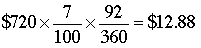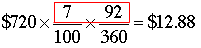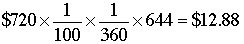SEARCH HOMEMath Central Quandaries & QueriesQuestion from Kenneth: Hello: I read about this method in an old business mathematics book and was curious about how it works to produce the correct answer. "Percent-day method is based on 360 days‚ time and 1% interest. Any interest rate multiplied by any number of days is equal to so many %-days." Example: 82 days at 5% equals 410%-days. "The rest of the calculation to find interest using his method involves multiplying the amount of interest at 1% by the ratio of %-days to 360." Here is an example calculation: $720 for 92 days at 7% = 7% X 92 days = 644%-days. 644/360 X$7.20(1% for 360 days) = $12.88 Can you explain the logic of this calculation? I can understand that days multiplied by percent equals %-days, but I do not understand how$\$720$ becomes $\$7.20$and why is (1% for 360 days) used? In other words, why does the method involve multiplying the amount of interest at 1% by the ratio of %-days to 360? What became of the 92 days? I thank you for your reply.Kenneth, For the example you give of$\$720$ for 92 days at 7% based on 360 days the conventional calculation would be to find 7% of $\$720,$which would be for 360 days and then multiply by 92/360 since you only used 92 of the 360 days. This would give. In the percent-day method the multiply the 7 and 92 firstto get. Since$\$720/100 = \$7.20$the resulting calculation is$\frac{644}{360} \times \$7.20 = \$12.88.\$

I hope this helps,
PennyMath Central is supported by the University of Regina and The Pacific Institute for the Mathematical Sciences.